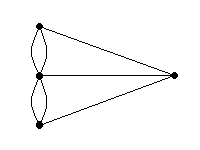# Traceability of Graphs

If a graph has no end vertices , you can ask the question: is it possible to trace over all the edges in the graph exactly once , without lifting your pencil? For instance, is the graph shown below traceable?The Swiss mathematician Leonhard Euler (a big name in the history of mathematics) solved this problem by reasoning as follows.

Every time you take your pencil through a vertex, you use two edges: one on the way in, and another on the way out. Vertices with odd degrees are going to cause problems: the only way to deal with these are to start tracing or end tracing there. So, a graph is not traceable if it has more than two vertices of odd degree.

By this theorem, the graph above is not traceable, since all four vertices have odd degree .

Euler actually proved the converse of the theorem as well: every graph with no end vertices and with two or fewer vertices of odd degree is traceable.